Mathematics

# Complete Guide: How to work with Negative Numbers in Abacus?

1.4k views

 1 Introduction 2 What is an Abacus? 3 Abacus Subtraction 4 Negative Numbers in Abacus 5 Conclusion 6 FAQs 7 External References

12 November 2020

## Introduction

25+75? 100

225+736? Hmm, 961.

225*736? Need to take the calculator out.

Managing large numbers in calculations has always troubled mankind and is also the reason for the invention of calculators. In here, we learn about the world's first calculator, the Abacus.

The abacus is the most ancient calculating device known. It has endured over time and is still in use in some countries.

The Abacus is a Latin word meaning sand tray. The term originated with the Arabic ‘abq’, which refers to dust or sand. In greek, this becomes ‘abax’ or ‘abakon,’ meaning a tablet. The abacus was used as a counting tool before the advent of the Arabic numeral system.

As one can imagine, how difficult it would be to count without numbers. There was a time when written numbers did not exist. The earliest counting device would have been the human fingers or toes. But for greater or bigger numbers, people would depend upon natural resources available to them, such as pebbles, seashells, etc.

Merchants and traders needed to maintain an inventory of the goods they bought and sold. When the Hindu-Arabic number system came into use, abaci ( plural of abacus) were adapted for place-value counting.

 📥 Complete Guide: How to work with Negative Numbers in Abacus? Download

## What is an Abacus?

An abacus or a counting board consists of a wooden frame, rods, and beads. Each rod represents a different place value—ones, tens, hundreds, thousands, and so on. Each bead represents a number, usually 1 or 5, and can be moved along the rods.

Addition and subtraction can easily be performed by moving beads along the wires of the abacus. The beads that slide along a series of wires or rods set in a frame to represent the decimal places.

The standard abacus can be used to perform addition, subtraction, division, and multiplication. It can also be used to extract square-roots and cubic roots. The beads are manipulated with either the index finger or the thumb of one hand.

The abacus is typically constructed of various types of hardwoods and comes in varying sizes. The abacus frame has a series of vertical rods on which several wooden beads can slide freely.

A horizontal beam separates the structure into two sections, known as the upper deck and the lower deck. One could call it a precursor to the modern-day calculator. Merchants commonly used it in Europe and the Arabic world.

In the modern world of computers and calculators, it is used by traders, merchants, etc. in many parts of the world. It is still used to teach the basics of arithmetic to children.

To know more, read Abacus from Basics.

## Abacus Subtraction

It is better to understand subtraction using an example. Let us begin with something simple and try to calculate $$102-78$$.

 Example 1

$$102-78=24$$

Step 1: Set 102 on rods ABC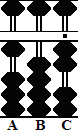Step 2: Subtract 7 from the tens rod B. Use the complement. Begin by subtracting 1 from hundreds rod A, then add the complementary 3 to rod B and so we are left with 32 on rods BC.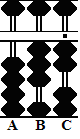Step 3: Subtract 8 from units rod C. Use the complement again. Begin by subtracting 1 from hundreds rod A, then add the complementary 2 to rod C, leaving the answer 24 on rods BC.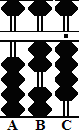Now, moving on to something that looks complicated but follows the same procedure for solving it.

 Example 2

$$22.3-2.8=19.5$$

Step 1: Designate rod B as the unit rod. Set 22.3 on rods ABC.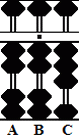Step 2: Subtract 2 from units rod B, leaving 20.3 on rods ABC.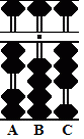Step 3: Subtract 8 from tenths rod C. Use the complement. Begin by subtracting 1 from units rod on B but here seems to be a problem. Rod B is empty. There's nothing there to subtract, so we have to go all the way over to rod A for help.

Step 4: Subtract 1 from tens rod A. Add 9 to rod B, then add the complementary 2 to rod C, leaving 19.5 on rods ABC.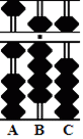Though it might look as though the last example is a bit of stretch, with practice, this can seem pretty easy. Suppose we want to look at something complicated we need to start solving examples such as $$78-102$$ or $$2.8-22.3.$$ Basically, we need to start addressing negative numbers on an abacus.

## Negative Numbers in Abacus

In the following examples, negative numbers can result when we subtract a larger number from a smaller one. Since there doesn’t exist a process of representing negative numbers on the abacus, expressing negative numbers is done through complementary numbers.

### Complementary Numbers at a Glance

To see negative numbers on a soroban, it is necessary to recognize complementary numbers quickly. From left to right, the rods below show the values 3, 42, and 286.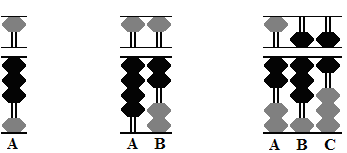Notice the grey beads. They form the basis for the complementary number, and to find the correct complementary number for each of the above, add plus 1 to the total value of grey beads.

In the first example of 3, the grey beads add up to 6. Plus 1 equals 7, the complement of 3 with respect to 10.

In the second example of 42, the grey beads add up to 57. Plus 1 equals 58, the complement of 42 with respect to 100.

In the third example of 286, the grey beads add up to 713. Plus 1 equals 714, the complement of 286 with respect to 1,000.

### Rule i) Always add plus 1 to the last value of the complementary number to find the right complement.

 Example

$$13 - 78 = -65$$

Step 1: Set 13 on rods DE with E acting as the unit rod.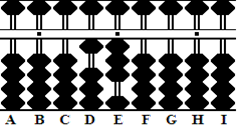Step 2: Subtract 78 from 13. As it stands, this is not possible, and this tells us we will have a negative result. On a soroban the way to solve this problem is to borrow. To give us something to borrow, set 1 on hundreds column C. This leaves 113 on rods CDE.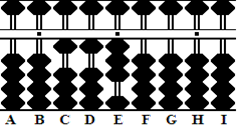Except for practice purposes, it is unnecessary to set the 1 on rod C, as shown in the above step. It is enough to do it mentally.

Step 3: and the answer: With 113 on CDE there is enough to borrow and complete the operation. Subtract 78 from 113, leaving 35 on DE. The negative answer appears in the form of a complementary number, as seen on the grey beads. Add plus 1 to the complementary number, and the final solution is -65.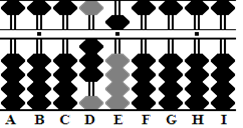### Rule ii) If the number being subtracted is too large, borrow from the rods on the left and continue the operation as if doing a normal subtraction problem.

 Example

Add 182 to the result in the above equation. $$[-65 + 182 = 117]$$

Step 1: The process of adding 182 to negative 65 is straightforward. Simply add 182 to the 35 already set on rods DE. This equals 217.

Step 2:  And the answer: Subtract the 100 borrowed in Step 2 of the previous example. This leaves 117 on rods CDE, which is the answer.

### Rule iv) Give back the amount borrowed if subsequent operations yield a positive answer.

 Example

$$19 - 72 - 7846 = -7,899$$

Step 1: Set 19 on DE with E acting as the unit.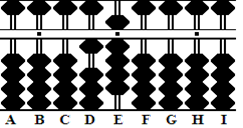Step 2: To subtract 72 from 19, borrow 1 from the hundreds column on rod C, leaving 119 on CDE

Step 3: Subtract 72 from 119, leaving 47 on rods DE. Interim, this leaves the complementary number 53 on rods DE.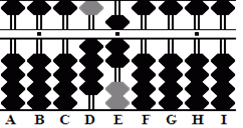In Step 2, the operation required borrowing 100 from rod D. In this next step, it will be necessary to borrow again, but borrowing a further 10,000 would bring the total to 10100. That would be wrong. Instead, borrow a further 9900 bringing the total amount borrowed to 10,000.

Step 4: To subtract 7846 from 47 on rods DE borrow 9900. This leaves 9947 on rods BCDE.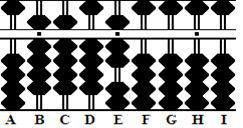### Rule v) If the operation requires borrowing again, only borrow 9s from adjacent rods. Remember that all complementary numbers on the soroban result from powers of 10. (10, 100, 1000, and so on.)

Step 5 and the answer: Subtract 7846 from 9947, leaving 2101 on rods BCDE. Once again, the negative answer appears in the form of a complementary number. Add plus 1 to the complementary number, and the answer is -7899.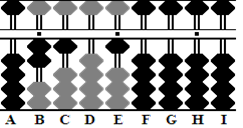Example

Subtract a further 31 from the result in the above equation.

$$[- 7899 - 31 = -7930]$$

Step 1 and the answer: Subtract 31 from 2101, leaving 2070 on rods BCDE. The complementary number shows 7920. Because rod E does not have a value, add plus 1 to the complement on rod D instead. This leaves the complementary answer -7930.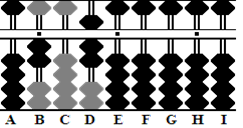### Rule vi) When adding 1 to the last digit of the complementary number, add only to the last rod with a value.

For more Examples and Practice questions, one can visit http://totton.idirect.com/abacus/pages.htm for detailed information on Abacus and many related techniques.

## Conclusion

Despite the abacus being ancient in its origin, it is still in use today. It has been a boon for the visually challenged as learning placement value and other calculations can be done by touch.

In many countries, the abacus is taught to early school goers as it has been seen that it helps subtends have a better understanding of numbers.

So, let’s recapitulate

• The abacus instrument is durable
• The abacus can be used to help young children learn numerical concepts.
• It helps in developing the skills at correctly manipulating beads on the counting tool.
• It builds an understanding of mathematical processes such as division, multiplication, subtraction, and addition.

In this article, we explored how we can use the concept of complementary numbers to represent negative numbers on the Abacus.

## Related Blogs

Abacus Blogs
Abacus Anatomy & Construction Abacus History
Abacus Level I Abacus Level II
Abacus Counting Abacus Techniques
Abacus Multiplication Abacus Division
Negative Numbers in Abacus Abacus Basics

Cuemath, student-friendly mathematics and coding platform, conducts regular Online Live Classes for academics and skill-development, and their Mental Math App, on both iOS and Android, is a one-stop solution for kids to develop multiple skills.

Check out the fee structure for all grades and book a trial class today!

## What is an abacus?

An Abacus is a manual aid for calculating which consists of beads that can be moved up and down on a series of sticks or strings within a usually wooden frame. The Abacus itself doesn't calculate; it's merely a device for helping a human being calculate by remembering what has been counted.

## Where was Abacus first used?

The Abacus (plural abaci or abacuses), also called a counting frame, is a calculating tool used in the ancient Near East, Europe, China, and Russia, centuries before the adoption of the written Arabic numeral system. The exact origin of the Abacus is still unknown.

## What are the advantages of using an abacus?

Mathematical skills lay a secure foundation for higher classes.

Abacus education improves the skills of

• Visualization (photographic memory)
• Concentration
• Listening Skills
• Memory, Speed
• Accuracy
• Creativity
• Self Confidence
• Self-Reliance resulting in Whole Brain Development

## Who invented the abacus?

Many studies have shown that no one in particular has made the abacus but many believe it was made in China. An adapted abacus, invented by Tim Cranmer, called a Cranmer abacus is still commonly used by individuals who are blind. A piece of soft fabric or rubber is placed behind the beads so that they do not move inadvertently.

## Where was the Abacus invented?

The type of Abacus most commonly used today was invented in China around the 2nd century B.C. However, Abacus-like devices are first attested from ancient Mesopotamia around 2700 B.C.!

Award-winning math curriculum, FREE for a year